# Synchronization Basics

## Timing and Synchronization

Timing is an essential element to all test, control, and design applications and should be a key consideration in any system. It defines the measurement capabilities of systems and is constrained by variables such as distance.

Timing and synchronization technologies allow the correlation or coordination of events in time and maximize the value the system provides. The end goal may be to time system events to a known standard (for example, sampling at a given frequency) or to time events relative to one another or in response to one another. In data acquisition, these events can be multiple samples or samples between multiple systems. Timing is important because it helps you coordinate or compare the acquired data signals with time, so you can relate the signals to each other.

## Terminology

The most basic components of a synchronized system are the clock and triggers. The clock provides the timing at which the samples are acquired or generated for the multiple signals that need to be synchronized. Triggers provide a starting point for the synchronized signals. For a better understanding of timing and synchronization, learn the following basic terms.

Event – An event is an occurrence of some significance. The terms event and trigger are almost synonymous in the discussion of timing and synchronization. When considering an event or trigger, think of a push button that starts some sort of device. The action of pushing the button triggers the device to start.

Trigger – A trigger is a hardware or software event that prompts an action, such as starting or stopping an acquisition. Figure 1 shows an example of a push-button trigger starting an action.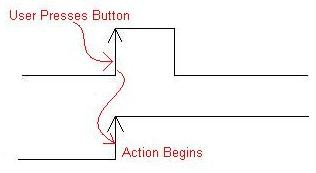Figure 1. Trigger

Timestamp – A timestamp is an event that is correlated to a specific time. The event, which can be a trigger signal or a data sample, is correlated to the device time when the event’s specified terminal changes state. Figure 2 shows an example of a trigger being timestamped to 12:00 PM.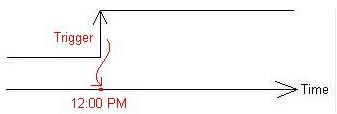Figure 2. Timestamp

Future time event (FTE) – An FTE is an event of some importance, such as a trigger, that is generated at a user-specified time. Figure 3 shows an example of an FTE configured to generate a trigger at 12:00 PM.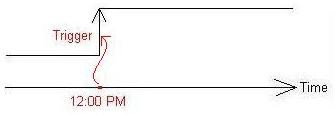Figure 3. Future Time Event

Clock – A clock is a periodic sequence of evenly spaced events. Figure 4 shows an example of a clock.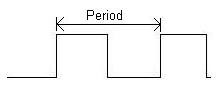Figure 4. Clock

There are two primary types of clocks: sample and reference.

Sample Clock – A sample clock controls the timing of the removal of samples from a data acquisition device. Figure 5 shows an example of a sample clock regulating at which time samples are taken from a signal.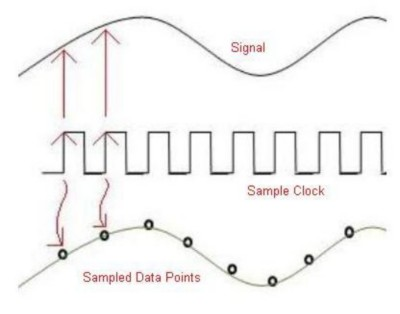Figure 5. Sample Clock

Reference Clock – A reference clock is a clock signal that is referenced by other systems’ clocks to derive their own clock signals. The derived clocks are multiplied or divided by certain values to obtain clocks that are slower or faster than the reference clock. Figure 6 shows an example of clocks derived from a reference clock.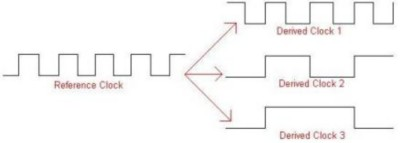Figure 6. Reference Clock

Master and Slave Devices – When creating synchronized measurement systems, you typically designate one device as a master and one or more other devices as slaves. The master device is the device that generates a signal or signals used to control all the measurement devices in the system. The slave devices receive control signals from the master device. Figure 7 shows the master/slave configuration.Figure 7. Master/Slave Configuration

## Asynchronous versus Synchronous

Asynchronous operations are actions that take place at an arbitrary time, without synchronization to a reference timer or clock. Figure 8 shows an example of an asynchronous acquisition. As seen in the graph, the sample trigger fires at arbitrary times, causing the data points to be unrelated to time. Examples of asynchronous operations are on-demand sampling and any data transfers where handshaking is used.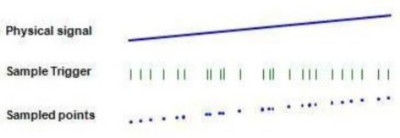Figure 8. Asynchronous Acquisition

Synchronous operations are when data is measured or generated at a constant rate using a shared periodic clock. Figure 9 shows an example of a synchronous acquisition.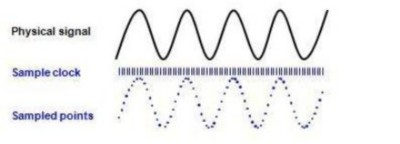Figure 9. Synchronous Acquisition

## Sources of Timing Error

Timing error describes how well you can measure or provide time. For example, if you measure a perfect 1 ms pulse and your measurement result is 1.003 ms, the 0.003 ms is your timing error in this measurement. When you report timing, timing error describes how well you provide the desired frequency. For instance, if you want to sample an analog voltage, you need a sample clock. Suppose you specify a 1 MHz sample clock while the actual sample clock frequency is 1.001 MHz. In this case, the 0.001 MHz frequency error represents the timing error.

Clock accuracy describes how well the actual frequency of the clock matches the specified frequency. Clock accuracy is described in parts per million (ppm). An accuracy of 5 ppm indicates that the mean frequency of the clock may be off by 5 Hz for every 1 MHz of its specified value. A 1 MHz clock with an accuracy of 5 ppm may have a frequency error of up to 5 Hz. A 2 MHz clock with the same accuracy may have a frequency error of up to 10 Hz.

Clock stability describes how well the clock frequency resists fluctuations. Factors that can cause the frequency to fluctuate include variations in temperature, time (aging), supply voltage, shock, vibration, and capacitive load that the clock must drive. Temperature is often the dominant factor that affects crystal oscillator stability.

The curve in Figure 10 represents the frequency of a 10 MHz clock over time. This clock is neither accurate nor stable. If you take the average value of the frequency, it is greater than 10 MHz. Furthermore, the value of the frequency fluctuates about a mean value over time.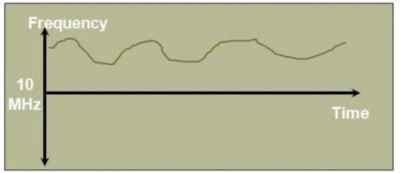Figure 10. Inaccurate, Unstable 10 MHz Clock

The curve in Figure 11 represents the frequency of a 10 MHz clock over time. If you take the average value of the frequency, it is very close to 10 MHz. Thus, this clock is accurate. However, the clock is not stable because the frequency fluctuates about its mean at approximately 10 MHz.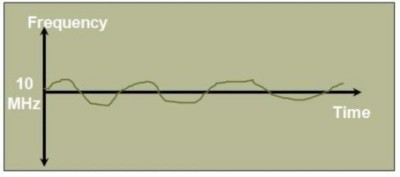Figure 11. Unstable, Accurate 10 MHz Clock

The curve shown in Figure 12 represents the frequency of a 10 MHz clock over time. This clock is stable because its frequency does not fluctuate. However, because its frequency is greater than 10 MHz, it is not accurate.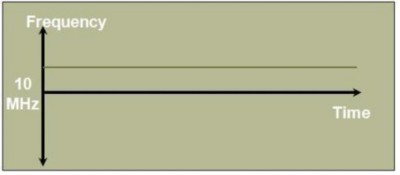Figure 12. Stable, Inaccurate 10 MHz Clock

The last curve, shown in Figure 13, represents the frequency of a 10 MHz clock over time. Its value is very close to 10 MHz and it shows very little or no fluctuations. Thus, this clock is accurate and stable.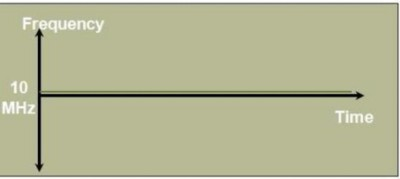Figure 13. Accurate, Stable 10 MHz Clock

Consider a system that has two clocks in two separate domains, as shown in Figure 14. If the clocks are started at exactly the same time, why do they drift apart from each other as time passes? Even identical clocks have small variances in frequency that, over time, add up to large differences and can cause the clocks to drift apart.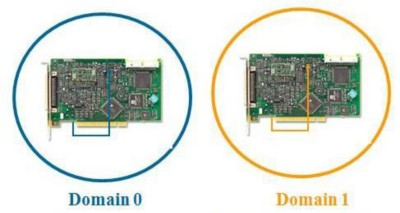Figure 14. Separate Clock Domains

Clock drift occurs when two instruments are acquiring data at different sample rates. Even though two instruments are set for the “same” sample rate – for example, two digitizers acquiring at 100 MHz – the real oscillators on each instrument run at different rates and therefore clock drift occurs. Figure 15 shows an example of clock drift.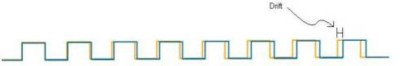Figure 15. Clock Drift

One solution to remedy clock drift is to have a common clock domain for all modules in a system. If you use the clock of one device as the clock for all devices, then you can eliminate clock drift. Figure 16 shows an example of a common clock domain.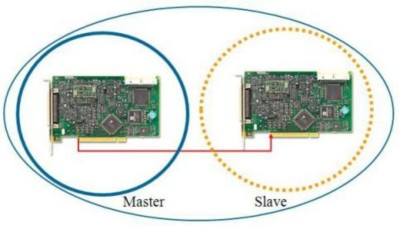Figure 16. Common Clock Domains

Clock skew is a phenomenon in synchronous circuits in which the clock or trigger signals arrive at different slave devices at different times. Skew can be caused by many different things, such as wire-interconnect length, temperature variations, variation in intermediate devices, capacitive coupling, material imperfections, and differences in input capacitance on the clock inputs of devices using the clock. As the clock rate of a circuit increases, timing becomes more critical and less variation can be tolerated if the circuit is to function properly. Figure 17 shows an example of clock skew.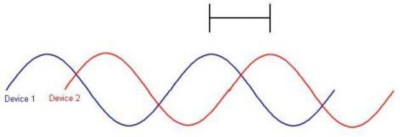Figure 17. Clock Skew

One solution to clock skew is ensuring that all clock signal paths are the same length. The PXI chassis backplane has signal lines, called star trigger lines, which are equal in length. Clock signals distributed on these lines arrive at their destinations at the same time, eliminating skew. Figure 18 shows an example of the PXI backplane’s star trigger lines.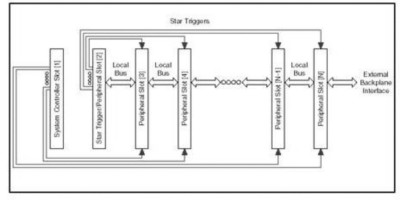Figure 18. PXI Backplane with Star Trigger Lines

Clock jitter, the deviation from the ideal timing of an event, is typically measured from the zero-crossing of a reference event or signal. Jitter usually results from crosstalk, electromagnetic interference, simultaneous switching outputs, and other regularly occurring interference signals. Because jitter varies over time, the measurements and quantification of jitter can range from a visual estimate on a scope in seconds to a statistical-based measurement such as one based on the standard deviation over time. Figure 19 shows an example of clock jitter.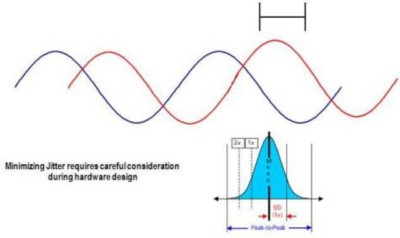Figure 19. Clock Jitter